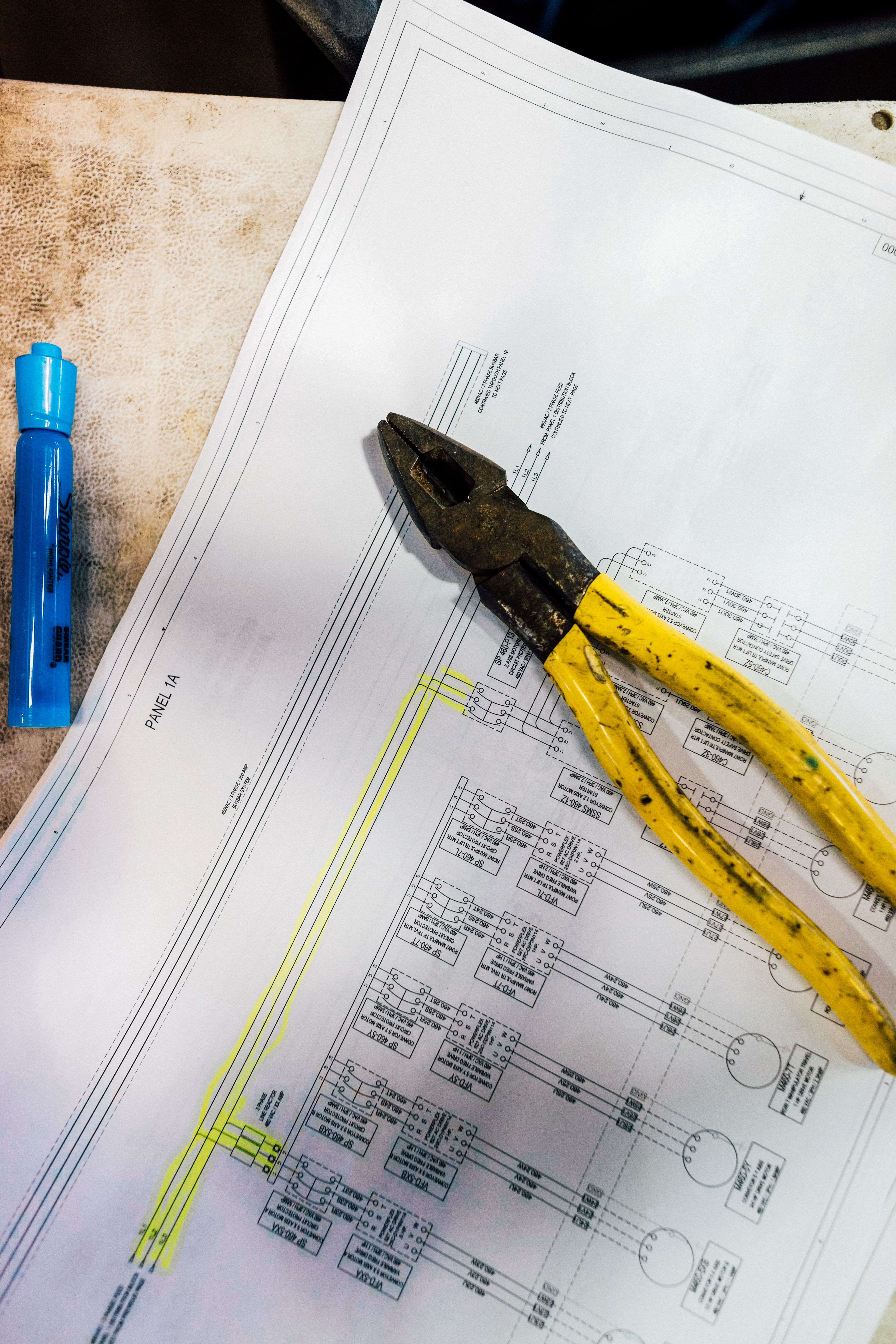# Is mechanical engineering math heavy?

Is mechanical engineering math heavy?

# Is mechanical engineering math heavy?Engineering is a field that requires a lot of problem-solving and analysis. One of the main aspects of engineering that students often worry about is the amount of math that is required. In this article, we will discuss whether mechanical engineering is math-heavy or not.

## FAQs

### What math courses are required for a mechanical engineering degree?

Regardless of whether it is mechanical, electrical, or civil engineering focused, engineering is a calculus-heavy program. The first circuits class you’ll take in this program requires Calculus 2 as a prerequisite. Other math requirements of the degree are calculus-based physics and differential equations.

### How much calculus is required for mechanical engineering?

Calculus is a critical tool for mechanical engineering. Students must master calculus to understand kinematics, dynamics, and thermodynamics—the main branches of mechanical engineering. Mechanical engineering students typically take 4-5 calculus courses. The first two courses are focused on differentiation, integration, integration techniques, and series. The last two courses address partial derivatives, multiple integrals, line integrals, Green’s theorems, Stoke’s theorems, and vector fields.

### What other math skills are required for mechanical engineering?

Trigonometry and linear algebra skills are also required for mechanical engineering. Trigonometry is frequently used in statics and dynamics courses, which are required courses in the mechanical engineering curriculum. Linear algebra is used in courses such as vibrations, control systems, and finite element analysis.## Why is math important in mechanical engineering?

Engineering is all about designing, building, and testing things. To do so effectively, you must have a thorough grasp of mathematical concepts. Mechanical engineers must apply calculus, statistics, and differential equations to perform mathematical modeling of physical elements like structures, fluids, and machines. Mechanical engineering projects require an extensive amount of math to design and evaluate complex mechanical systems.

## What are some resources you can use to improve your math skills in mechanical engineering?

There are many resources available to mechanical engineering students who want to improve their math skills. Firstly, finding a support group is essential. All universities have a math tutoring center. There are YouTube videos and websites like MIT OpenCourseWare that can assist students in learning engineering math concepts.## Conclusion

Math is an essential element of mechanical engineering, and students should take every opportunity to improve their math skills. Mechanical engineers must apply calculus, statistics, and differential equations to perform mathematical modeling of physical elements. It is vital for engineers to have an in-depth knowledge of mathematical concepts to analyze and design complex mechanical systems successfully.